### Home > CC1 > Chapter 8 > Lesson 8.2.1 > Problem8-74

8-74.
1. Read each question below and decide if it is a statistical question. If possible, reword the question so that it is a statistical question. Homework Help ✎

1. How tall is the Empire State Building?

2. How much milk do Americans drink each day?

3. What is the price of an oil change in Seattle, Washington?

4. What size shoe do you wear?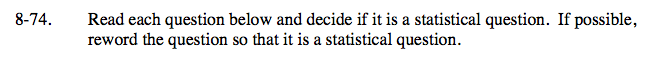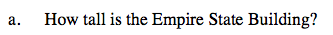How many answers are there to this question?
(Statistical questions must have multiple answers.)

This is not a statistical question, but there is no way to rewrite this question.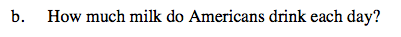Is this question asking for one number or for multiple numbers?

This is not a statistical question because it is asking for one number. Instead, ask a group of Americans how much milk each one of them drinks per day.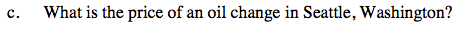Is this asking for one answer or for multiple answers?
(Do all of the mechanics charge the same amount for an oil change?)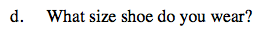How many answers are there to this question?
(Do you have multiple shoe sizes?)